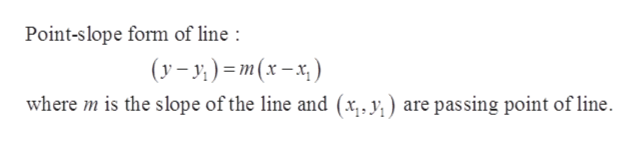# Find f'(x) and find the equation of the line tangent to the graph of f at the indicated value of xf(x) x(3-x), x2f'(x)The equation of the line tangent to the graph of f at x 2 is yEnter your answer in each of the answer boxes.hp

Question
12 viewshelp_outlineImage TranscriptioncloseFind f'(x) and find the equation of the line tangent to the graph of f at the indicated value of x f(x) x(3-x), x2 f'(x) The equation of the line tangent to the graph of f at x 2 is y Enter your answer in each of the answer boxes. hp fullscreen
check_circle

Step 1

Given:

Step 2

Formula used:help_outlineImage TranscriptionclosePoint-slope form of line (y-y)m(x-x where m is the slope of the line and (x,. y) are passing point of line fullscreen
Step 3

In this case...

### Want to see the full answer?

See Solution

#### Want to see this answer and more?

Solutions are written by subject experts who are available 24/7. Questions are typically answered within 1 hour.*

See Solution
*Response times may vary by subject and question.
Tagged in

### Calculus